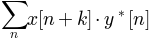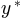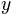matplotlib.axes.Axes.xcorr¶

Axes.xcorr(x, y, normed=True, detrend=<function detrend_none>, usevlines=True, maxlags=10, *, data=None, **kwargs)[source]

Plot the cross correlation between x and y.

The correlation with lag k is defined as, whereis the complex conjugate of.

Parameters: x : sequence of scalars of length n y : sequence of scalars of length n detrend : callable, optional, default: mlab.detrend_none x is detrended by the detrend callable. Default is no normalization. normed : bool, optional, default: True If True, input vectors are normalised to unit length. usevlines : bool, optional, default: True If True, Axes.vlines is used to plot the vertical lines from the origin to the acorr. Otherwise, Axes.plot is used. maxlags : int, optional Number of lags to show. If None, will return all 2 * len(x) - 1 lags. Default is 10. lags : array (length 2*maxlags+1) lag vector. c : array (length 2*maxlags+1) auto correlation vector. line : Artist added to the axes of the correlation LineCollection if usevlines is True Line2D if usevlines is False b : Line2D or None Horizontal line at 0 if usevlines is True None usevlines is False linestyle : Line2D property, optional Only used if usevlines is False. marker : string, optional Default is 'o'.

Notes

The cross correlation is performed with numpy.correlate() with mode = 2.

Note

In addition to the above described arguments, this function can take a data keyword argument. If such a data argument is given, the following arguments are replaced by data[<arg>]:

• All arguments with the following names: 'x', 'y'.

Objects passed as data must support item access (data[<arg>]) and membership test (<arg> in data).